# How to Create and Edit a Cut Section?

VMoveCAE provides the ability to create cut-sections.

This module details the steps for creating cut-sections.

In this example beam.rst file provided in the samples directory is used.

2. Click on the Add Cut-Section icon to create cut-sections.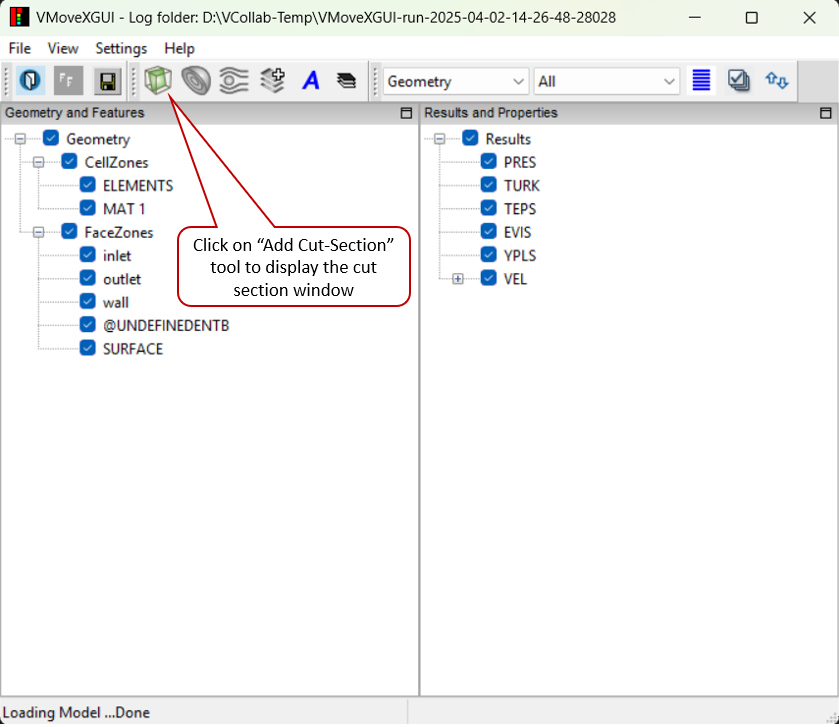This brings up the cut-section window.

3. Add a cut-section: A cut-section can be added by entering an equation and clicking Add. The

cut-section equation needs to be in the form of “AX+BY+CZ=D”.

In this example “X=50” has been entered.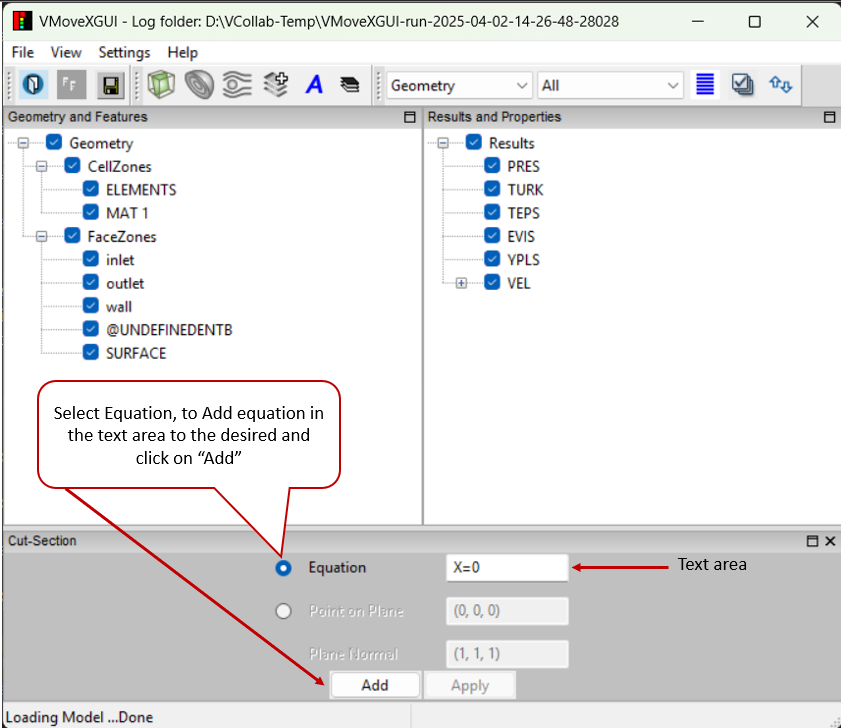The new item gets added to the Cut-Sections tree under found under Features.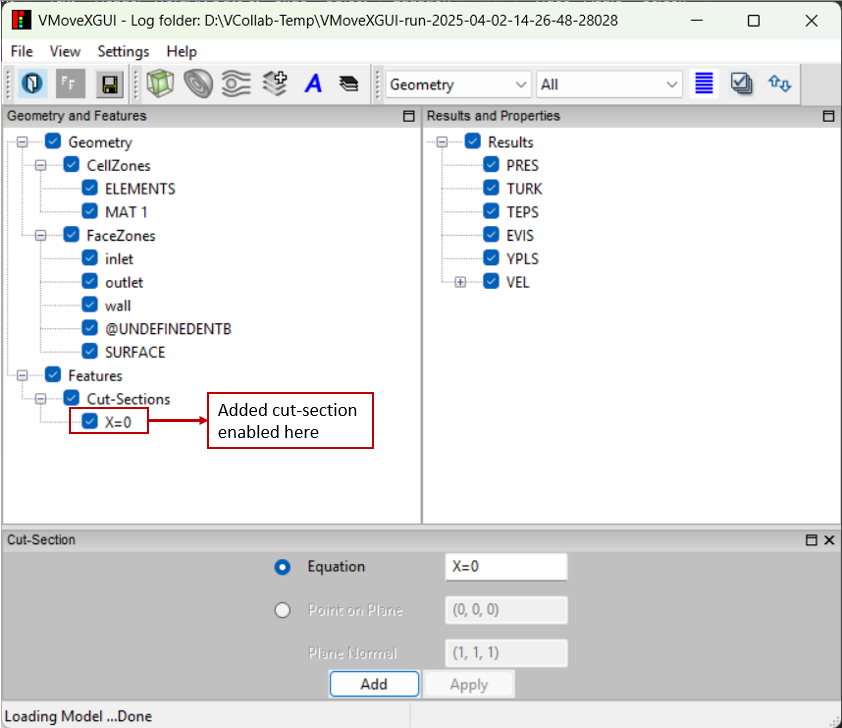4. More cut-sections can be added in a similar way. For example, another cut-section with “Y=5” can be added.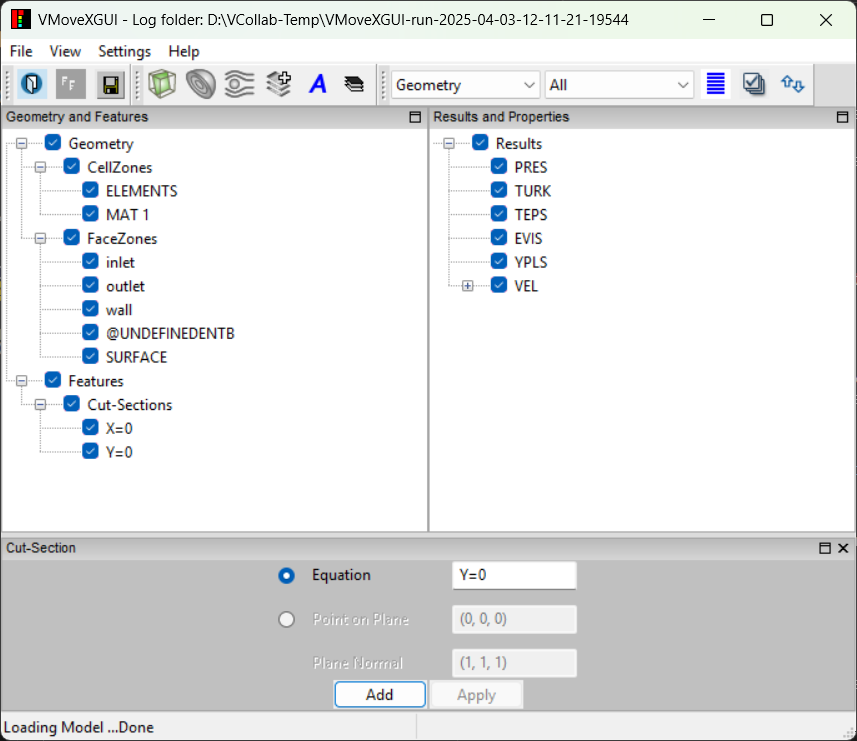An existing cut-section can be changed by changing its Plane Equation.

Select the cut-section “Y=5” and change the value to “X-2.4Y+1.6Z=80”.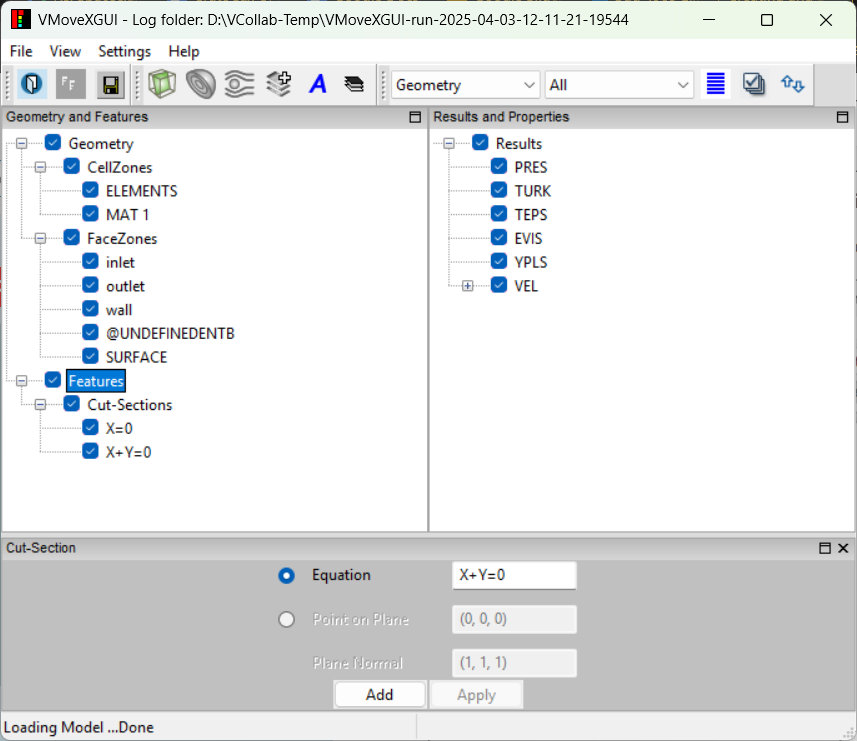5. Proceed to translate to CAX file by clicking on Save CAX icon. The output CAX file can be opened in VCollab Professional or VCollab Presenter to visualize the file cut-sections. If any cut-section is out of bounds and not cutting the geometry then VMoveCAE ignores it and the cut-section will not be created.

6. Generation of multiple parallel cut-sections Multiple parallel cut-sections can be specified and created in one go with VMoveCAE. For this, specify a range and number of cut-sections on the right side of the expression instead of a value. If no limit is specified by the user, VMoveCAE automatically computes the domain boundaries and uses them as limits.

Examples:

1. A plane equation of “Y = 0:10:5” results in the generation of five cut-sections for “Y=1”, “Y=3”, “Y=5”, “Y=7” and “Y=9”. Zero represents the lower limit, ten represents the higher limit and the five represents the number of cut-sections.

2. A plane equation of “Y = :: 5” creates five equally spaced cut-sections along the Y-axis.

3. The user can also specify only one of the limits, letting VMoveCAE compute and use the domain limit for the other limit. For example, “Y=:10:5” uses the domain minimum of the Y-coordinate value as the lower limit.